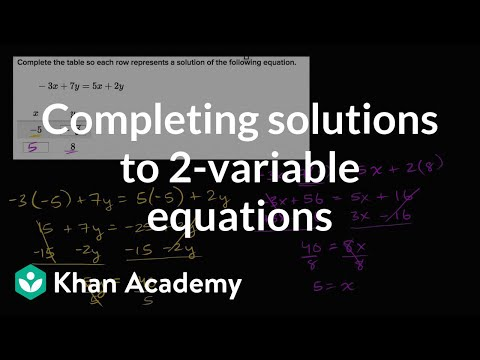# Systems of equations with elimination: -3y + 4x = 11 and y + 2x = 13 (Full video)

Description: Sal demonstrates how to solve the system of equations -3y + 4x = 11 and y + 2x = 13 using the elimination method. Maybe we can add these two equations together to get some variables to cancel out. But if we just add y and negative 3y, those won't cancel out. But maybe we can scale one of these equations, so that we will get some cancellation.

### Other videos you might be interested in### Solving Systems of Equations by Substitution (Full video)

#### Student Achievement Partners